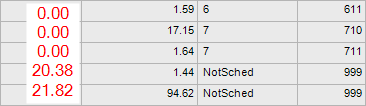# BAQ Conditional Sum or partial sum expression for column in table

I’m just not sure how to translate this into an expression for epicor or where I should be placing this logic.

I’m creating a BAQ to return a table of all jobs that follow certain criteria. I have the table returning correctly, however, we have a column that we use to track how many days away from being scheduled for production that any given job number is.

This is a BAQ and dashboard that is basically replacing an old excel spreadsheet. The Column 0 is originally in excel as the SUM of all Column 1 where Column 2 is scheduled and column 4 is smaller than current rows Column 3.

Pseudocode: if (current.col == NotSched) then current.col = sum(allrows.col1 where allrows.col3 < current.col3)

Quick example,It is using some UD fields attached to JobOper.

Use a Case statement to calculate that out in the BAQ.

Case
When Col3 = “NotSched” Then calculation
Else 0
End

I was thinking along these lines but I keep getting BPM003 “Expression should contain only one statement”. Is there a place I should be putting this expression besides in a bpm? Pretty new to Epicor right now.

Do a calculated field in the BAQ you created.

Wow I totally forgot about calculated fields!